Increasing and Decreasing Functions - IIT JEE

Monotonic Function

Increasing and decreasing functions can be easily explained with the help of derivatives as it is one of the most important applications of derivatives. Derivatives are generally used to identify whether the given function is increasing or decreasing at a particular interval of time. This increase and a decrease of the function enhance the appropriateness of the rate of change of quality obtained by the function. The increase and decrease of the function can be easily explained with the help of the following graph as the name suggests, increase in a function occurs when the line of the graph moves upwards while the decrease in a function occurs when the line of the graph moves downward, as in the following graph.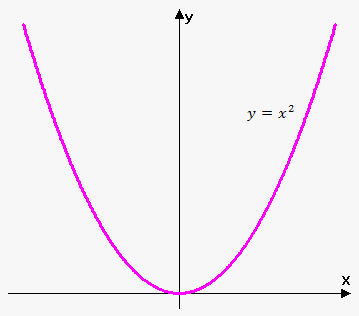If we are moving from left to right in the above shown graph then, starting from left the curve is moving downwards as the value of y is decreasing and the value of x is also decreasing at a given interval of time, so this is called as increasing function. When we move to the right side of the graph, the curve is increasing as both the values of x and y are increasing with the given interval of time and known as the decreasing function.

Increasing Functions:

The function which is increasing at a given interval of time is called an increasing function. The function of y = f(x) involves an increasing function having two points of intervals (x1 and x2) such that x1 < x2 with the functional inequality of f(x1) ≤ f(x2), then the function can be said to be an increasing function. In this inequality, some part of the increasing function remains equal with both the functions making it differ from strictly increasing function. While strictly increasing function involves that function which does not get equal to both the axis in between the increasing process of the function at the given interval of time. The strictly increasing function for the fixed interval of time having the intervals of x1 and x2 can be stated as f(x1) < f(x2). This increasing, as well as strictly increasing functions, can be easily shown on a graph with the help of the figures shown below;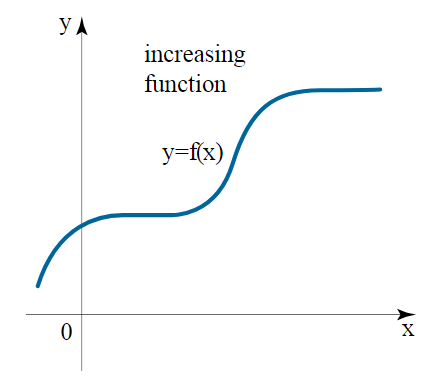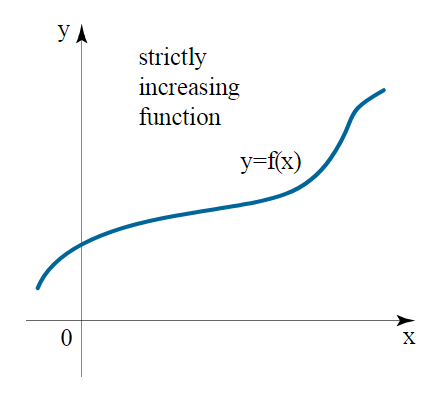Increasing function Strictly increasing function

Decreasing functions:

The functions which are decreasing at the given interval of time are called as decreasing functions. The function y = f(x) shows that the function has the interval points of x1 and x2 through which a function can be decreasing or strictly decreasing at the given interval of time. The function which is decreasing holds the unique inequality of f(x1) ≥ f(x2). This inequality of f(x1) ≥ f(x2) makes it unique as the line of graph remains equal for both the axis in a particular interval of time, making it different from strictly decreasing function. This strictly decreasing function can be defined as the function which strictly holds the inequality and does not have the same value for both the axis at a given interval of time and shows the function as f(x1) > f(x2). The main difference between the strictly decreasing and decreasing function is that at any interval of time the function does not remain the same in strictly decreasing type function. These decreasing types of function can be easily explained with the help of the graph as shown in the following figures;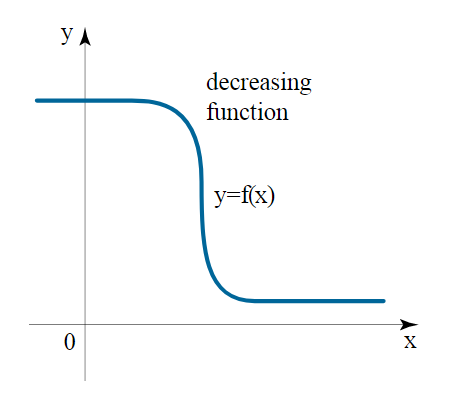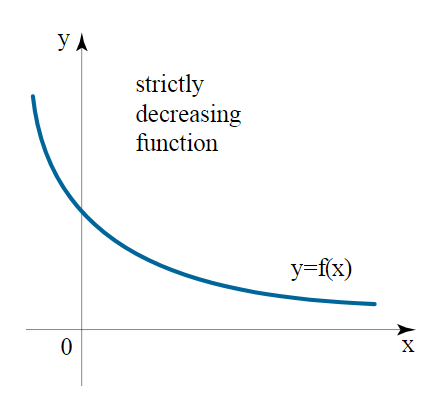Decreasing function Strictly decreasing function

Steps involved in calculating the increase and decrease intervals:

There are some steps involved in the process of finding the intervals of increasing and decreasing function, are as follows:

• • Firstly, the given function is differentiated with respect to the constant variable.

• • Then the first derivative is solved in the form of an equation to provide the value of x i.e. f’(x) = 0.

• • After solving the equation of the first derivative and the points of discontinuity we get the open intervals with the value of x, through which the sign of the intervals can be taken into consideration.

• • The points of interval formed are compared to find the sign in their first derivatives. If the sign of the interval in their first derivative form gives more than 0, then the function is said to be increasing in nature, while if the sign of the intervals in their first derivative form gives less than 0, then the function is said to be decreasing in nature.

• • Finally, we get increasing as well as the decreasing intervals of the function.

• There is a test through which the nature of the function can be identified which is known as the first derivative test. Through the first derivative test we can easily find whether the function is monotonic in nature or not.

Monotonic Function:

The functions which are differentiable at the given interval (a, b) of time and are included in any of the four categories which are increasing function, strictly increasing function, decreasing function or strictly decreasing function are called as monotonic functions. A function which includes df/dx = 0 is constant at that given interval of time. This monotonic nature of the function can be further explained in the first derivative test.

First Derivative Test:

As explained above, the nature of the function can be determined with the help of this test, as it varies with the value of the derivative of the function. The derivative of the function with respect to the independent behavior of the function shows different behaviours with the different values which are as follow;

• • If df/dx ≥ 0, for all the value of x with the given interval of time (a, b), make it the increasing function for all the value of f(x) at the given interval (a, b).

• • If df/dx ≤ 0, for all the value of x with the given interval of time (a, b), make it the decreasing type of function at the given interval (a, b).

• The strict inequalities depend on the monotonic nature in the first derivative test which can be shown mathematically as,
• • If df/dx > o then the function shows monotonic nature with the strict inequality of strictly decreasing function.

• • If df/dx < 0 then the function shows monotonic nature with the strict inequality of strictly increasing function.

• Proof of the first derivative test:

This test is done to show the increasing and decreasing nature of the derivative of the function. In the test of the first derivative, the function which is greater than or equal to 0 is called an increasing function with respect to the given interval of time (a, b). It can be expressed as if df/dx = 0 at the intervals (a, b) is said to be increasing in nature. If the derivative of the given function is
df/dx = Δx → 0 [f(x+Δx) – f(x)]/ Δx

If Δx > 0, then the RHS will be greater than 0 making the value of df/dx > 0 only if the value of the numerator on the RHS of the equation is greater than 0. Through this information, we can get f(x + Δx) ≥ f(x), which shows that the given function is greater than 0 and is stated as an increasing function.

Similarly, in the case of decreasing function, we let the value of Δx < 0, through which we get the value of df/dx less than 0 making the function decreasing in nature. Hence, through this process, first derivative test is proved to show the nature of the function, whether it is increasing or decreasing in nature.

The functions which are increasing, strictly decreasing, decreasing and strictly decreasing in nature at a given interval of time can be easily explained with the help of the following example:

If the curve of sin x at (0, π/4) and (π/4, π/2) is given and the nature of the curve is to be find out, then we can see the following solution to find the nature of the curve;

Solution:
First, we need to calculate the value of the first derivative of the given function (sin x), we get;
d (sin x) / dx = cos x
This derivative of the function sin x at (0, π/4) and (π/4, π/2) can be shown on the following graph;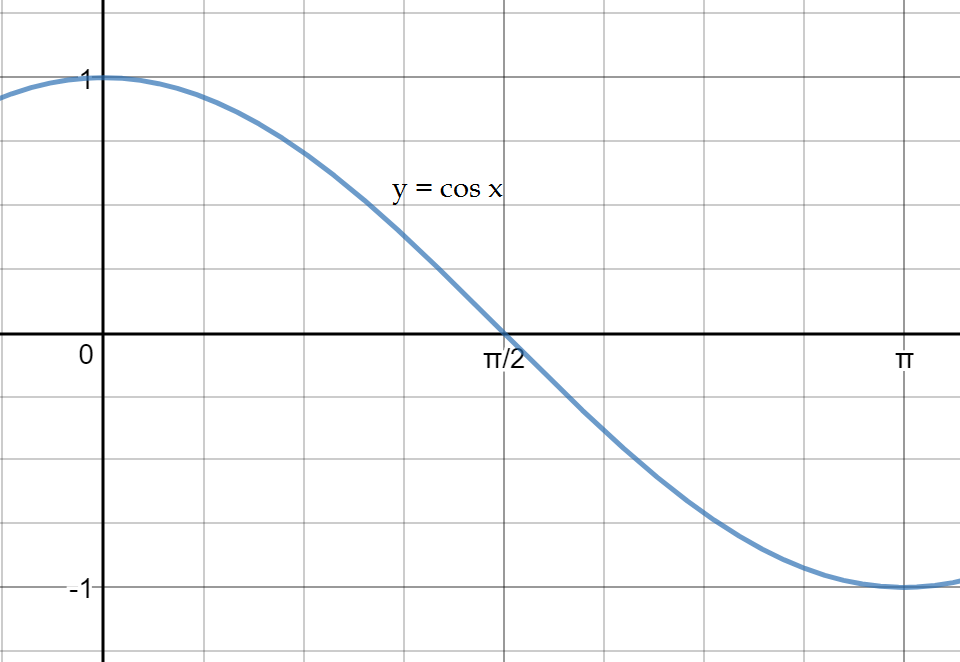From the above shown graph, we can easily conclude the following points:

• • For the function x ∈ (0, π/4), cos x > 0 i.e. d (sin x )/ dx > 0, which shows that the function is strictly increasing in nature as the value of the first derivative of the function is strictly greater than 0 at the interval (0, π/4).

• • For the function x ∈ (0,π/4), cos x < 0 i.e. d (sin x )/ dx < 0, which shows that the function is strictly decreasing in nature as the value of the first derivative of the function is strictly less than 0 at the interval (π/4, π/2).

• • Hence, through the above information, we can easily conclude the intervals at which the function is strictly increasing and strictly decreasing in nature.